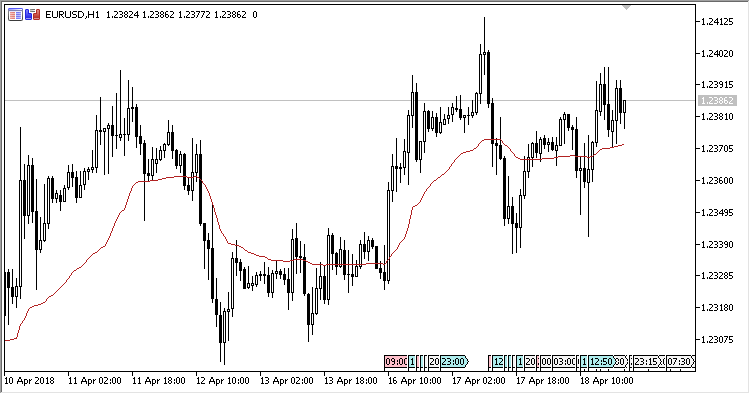# KAMA – indicator for MetaTrader 5

The Kaufman Adaptive Moving Average is a version of the adaptive moving average based on the exponentially smoothed moving average combined with the original methods of detecting and applying volatility as a dynamically changing smoothing constant.

Indicator named Adaptive Moving Average was developed by Perry J. Kaufman and primarily presented in his book titled Smarter Trading: Improving Performance in Changing Markets in 1995.

The indicator has two input parameters:

• Period – calculation period;
• Applied price – price used for calculations.

Calculations:

`KAMA[i] = KAMA[i-1] + sc * (Price[i] - KAMA[i-1])`

where:

```sc = (er * 0.6015 + 0.0645) * (er * 0.6015 + 0.0645),
er = Abs(Price[i] - Price[i-Period+1]) / Sum1, and
Sum1 = Sum(Abs(Price[i] - Price[i-1])) from (i-Period+1) to i
```